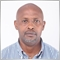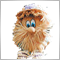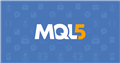# converting bollinger code from MT4 to MT5!336

Hi Community! Hope all's well!! Just slightly struggling with converting a piece of code(Bollinger Band and MA of the same) from back in the day in MQL4 to MQL5. Will be highly appreciated. Thanks much!!
```  RefreshRates();
// GETTING THE AMOUNT OF ALL BARS OF THE CHART
int IBARSA = iBars(NULL,A_TF);
//---- Checking whether the bars number is enough for further calculation
if(IBARSA < A_MA)
return(0);
// INDICATOR BUFFERS EMULATION
if(ArraySize(BandWidthA) < IBARSA)
{
ArraySetAsSeries(BandWidthA, false);
ArraySetAsSeries(MainA, false);
ArraySetAsSeries(PercentA, false);
//----
ArrayResize(BandWidthA, IBARSA);
ArrayResize(MainA, IBARSA);
ArrayResize(PercentA, IBARSA);
//----
ArraySetAsSeries(BandWidthA, true);
ArraySetAsSeries(MainA, true);
ArraySetAsSeries(PercentA, true);
}
// INSERTION OF A STATIC INTEGER MEMORY VARIABLE
static int IndCountedA;
//----+ Insertion of integer variables and GETTING ALREADY CALCULATED BARS
int A, MaxBarA, barA, counted_barsA = IndCountedA;
//---- checking for possible errors
if(counted_barsA < 0)
return(-1);
//---- the last calculated bar must be recalculate
if(counted_barsA > 0)
counted_barsA--;
//----+ REMEMBERING THE AMOUNT OF ALL BARS OF THE CHART
IndCountedA = IBARSA - 1;
//---- defining the number of the oldest bar,
//---- starting from which new bars will be recalculated
A = IBARSA - counted_barsA - 1;
//---- defining the number of the oldest bar,
//---- starting from which new bars will be recalculated
MaxBarA = IBARSA - 1 - A_MA;
//---- initialization to zero
if(A > MaxBarA)
{
A = MaxBarA;
for(barA = IBARSA - 1; barA >= 0; barA--)
{
BandWidthA[barA] = 0.0;
MainA[barA] = 0.0;
PercentA[barA]=0.0;

}
}
//------MAIN CYCLE
//---------------------1
for(int a = IBARSA-1; a >= 0; a--)
{
double UpperBandA = iBands(NULL,A_TF,A_MA,Deviation,Shift,2,MODE_UPPER, a);
double LowerBandA = iBands(NULL,A_TF,A_MA,Deviation,Shift,3,MODE_LOWER, a);
double MiddlBandA = iBands(NULL,A_TF,A_MA,Deviation,Shift,4,MODE_MAIN,  a);
double DenominatA = UpperBandA - LowerBandA;

if( MiddlBandA == 0.0 ) { MiddlBandA = 0.00001; }
if( DenominatA == 0.0 ) { DenominatA = 0.00001; }

BandWidthA[a] = ( UpperBandA - LowerBandA ) / MiddlBandA;
PercentA[a] = (Close[a]-LowerBandA)/DenominatA;

//----
}

while(A>=0)
{
//----
MainA[A]  = iMAOnArray(BandWidthA,0,A_MAMA,Shift,MODE_SMA, A);
//----
A--;
}
A_PerCent=PercentA;
double A_Diff=BandWidthA-MainA;
Print(" A Diff strength,,,   ",A_Diff);```Moderator
246806

Please read the Help - in MQL5, the indicator handle needs to be created ALL ONCE !!! And this is done, as a rule, in OnInit ()!336

Vladimir Karputov:
Please read the Help - in MQL5, the indicator handle needs to be created ALL ONCE !!! And this is done, as a rule, in OnInit ()!

Hey Vladimir,,, your help is quite appreciated. Quick question as follow up,,, once handle is created at OnInit(),,, how much of the code in the main body will i need to alter. Can i just leave it as is once created at OnInit(),,,, asking because the MODE_UPPER, MODE_LOWER and MODE_MAIN are not recognised!! Kindly. Thanks!336

Also Vladimir,,, wouldnt i need to do something about the iMAOnArray???? Thanks!!Moderator
246806

An example of creating an iBands indicatorDocumentation on MQL5: Technical Indicators / iBands
• www.mql5.com
iBands - Technical Indicators - MQL5 Reference - Reference on algorithmic/automated trading language for MetaTrader 5336

Vladimir Karputov:
An example of creating an iBands indicator

Thanks336

Kenneth Njuguna:

Thanks

Hi Vladimir,,,, first off thank you much for your help so far,,,  I have managed to figure out most of the conversion,,,, BUT for 1 piece,,,, how to calculate the moving average for the BandWidth Array,,

```  while(L>=0)
{
//----
MainL[L]  = iMAOnArray(BandWidthL,0,L_MAMA,Shift,MODE_SMA, L);
//----
L--;
}```

What can i use in MQL5/MT5 for the same functionality ??? Kindly!!!